# - Resources x Give Up? Hint Calculate the molarity of the two solutions. Thir first solution...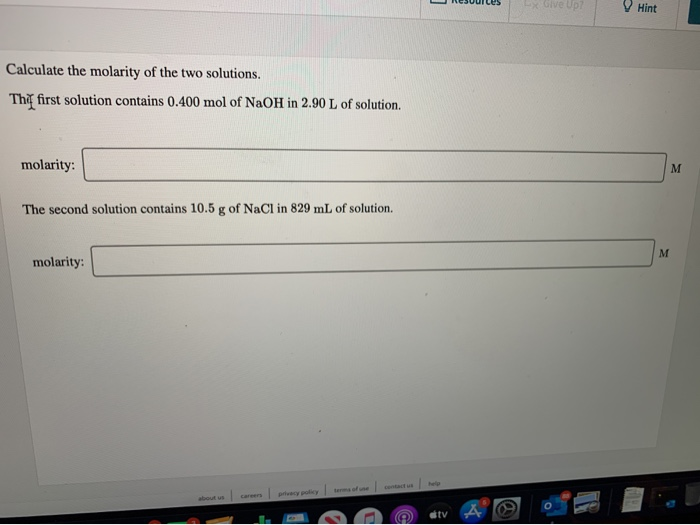- Resources x Give Up? Hint Calculate the molarity of the two solutions. Thir first solution contains 0.400 mol of NaOH in 2.90 L of solution. molarity: The second solution contains 10.5 g of NaCl in 829 mL of solution molarity: terms of use contact us help bus car precy policy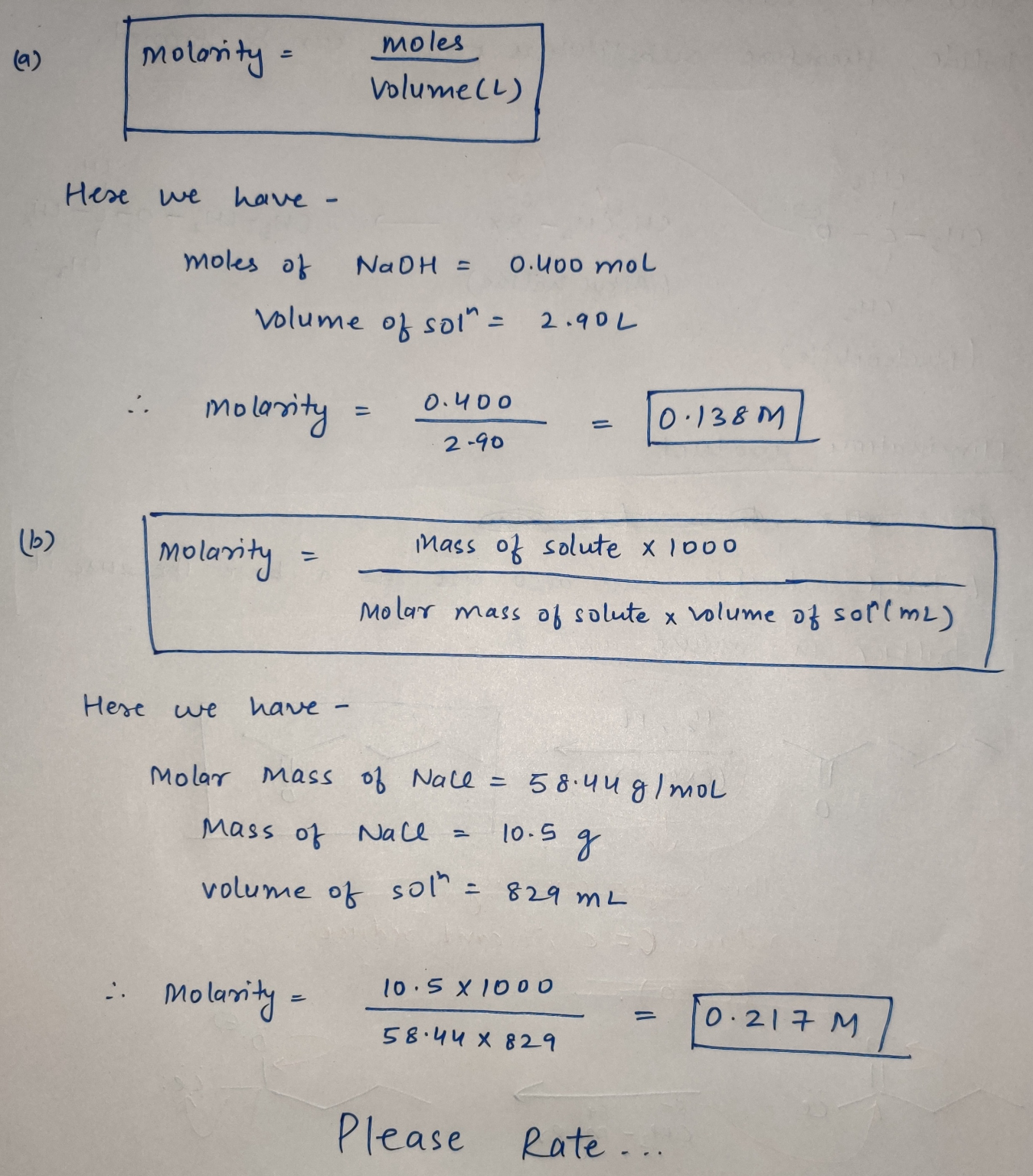#### Earn Coin

Coins can be redeemed for fabulous gifts.

Similar Homework Help Questions
• ### Calculate the molarity of the two solutions. The first solution contains 0.500 mol of NaOH in...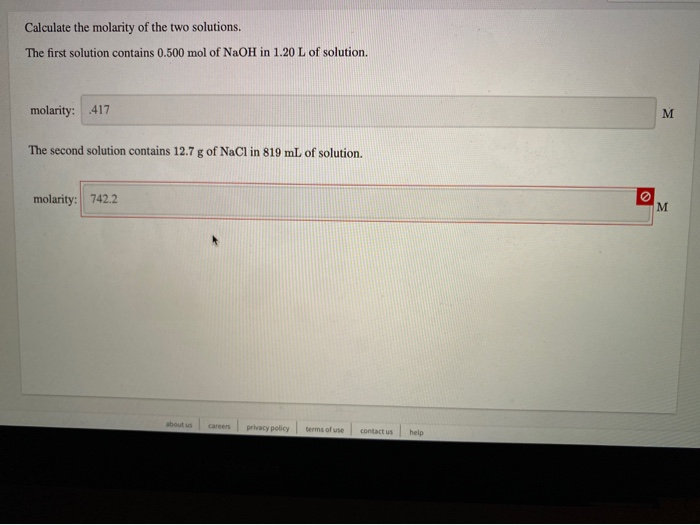Calculate the molarity of the two solutions. The first solution contains 0.500 mol of NaOH in 1.20 L of solution. molarity: 417 The second solution contains 12.7 g of NaCl in 819 mL of solution. molarity: 742.2 about us Careers privacy policy terms of use contact us help

• ### Calculate the molarity of the two solutions. The first solution contains 0.500 mol of NaOH in...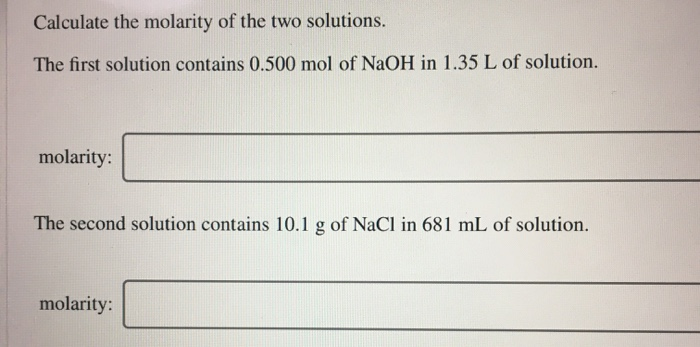Calculate the molarity of the two solutions. The first solution contains 0.500 mol of NaOH in 1.35 L of solution. molarity: The second solution contains 10.1 g of NaCl in 681 mL of solution. molarity:

• ### Calculate the molarity of the two solutions. The first solution contains 0.650 mol of NaOH in...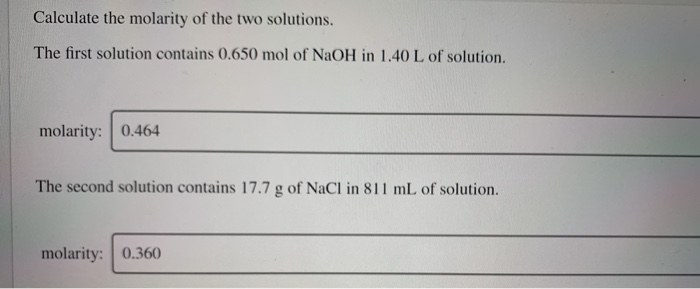Calculate the molarity of the two solutions. The first solution contains 0.650 mol of NaOH in 1.40 L of solution. molarity: 0.464 The second solution contains 17.7 g of NaCl in 811 mL of solution. molarity: 0.360

• ### Calculate the molarity of the two solutions. The first solution contains 0.450 mol of NaOH in 2.00 L of solution. molar...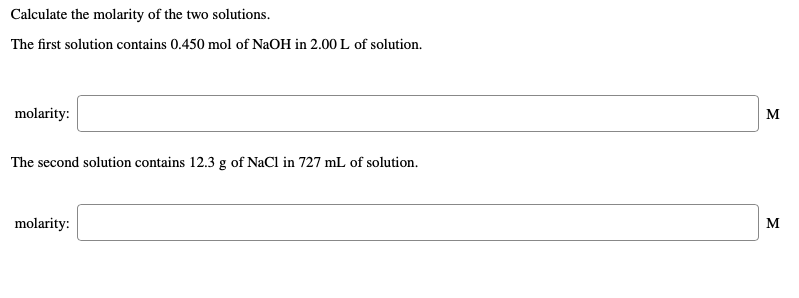Calculate the molarity of the two solutions. The first solution contains 0.450 mol of NaOH in 2.00 L of solution. molarity: The second solution contains 12.3 g of NaCl in 727 mL of solution. molarity:

• ### Calculate the molarity of the two solutions. The first solution contains 0.650 mol of NaOH in 1.40 L of solution. m...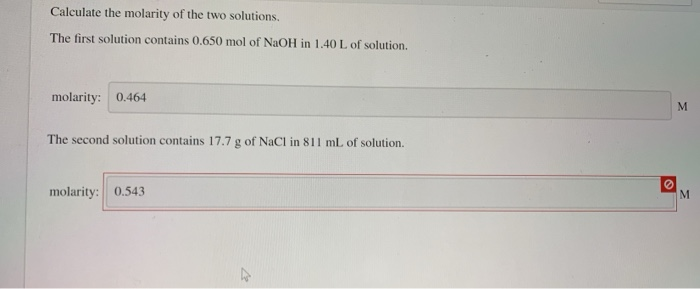Calculate the molarity of the two solutions. The first solution contains 0.650 mol of NaOH in 1.40 L of solution. molarity: 0.464 The second solution contains 17.7 g of NaCl in 811 mL of solution. molarity:

• ### Calculate the molarity of the two solutions. The first solution contains 0.550 mol of NaOH in...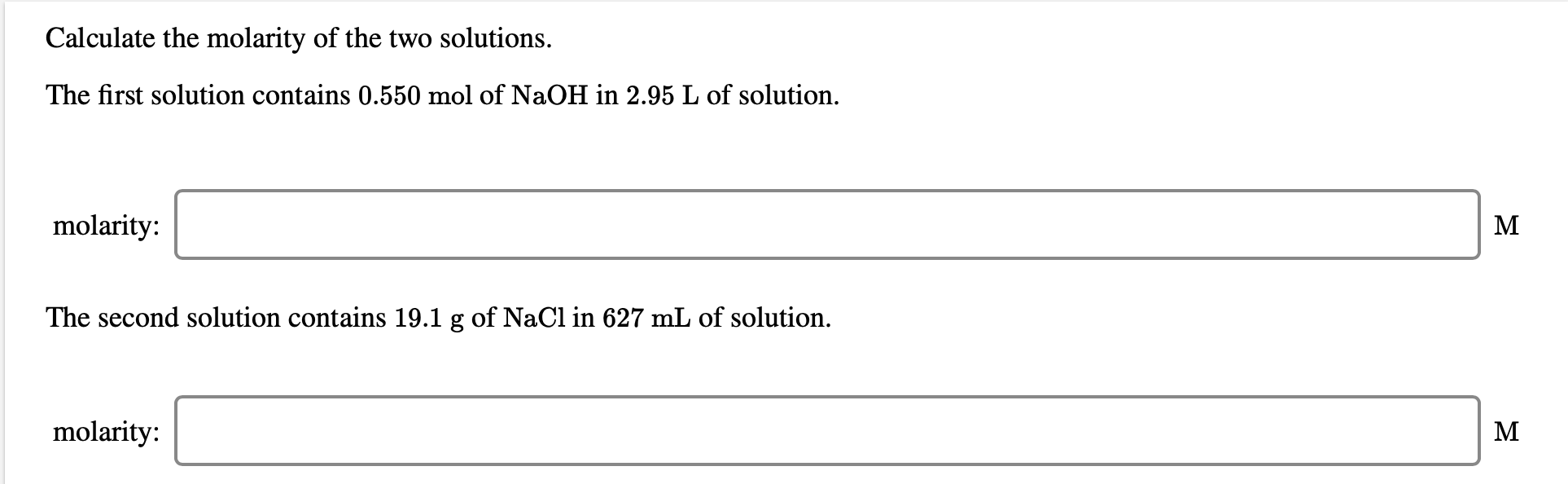Calculate the molarity of the two solutions. The first solution contains 0.550 mol of NaOH in 2.95 L of solution. molarity: The second solution contains 19.1 g of NaCl in 627 mL of solution. molarity: Calculate the molality of a solution formed by adding 7.00 g NH,Cl to 14.0 g of water. molality: m NH C1 What is the mole fraction of solute in a 3.69 m aqueous solution? Xsolute = 0.06 An aqueous sodium acetate, NaC,H,O, , solution is...

• ### Calculate the molarity of the two solutions. a) 0.350 mol of NaOH in 2.35 L of...

Calculate the molarity of the two solutions. a) 0.350 mol of NaOH in 2.35 L of solution. b) 13.3 g of NaCl in 811 mL of solution.

• ### 700/2400 Up Hint ment Score: Resources Check Answe tion 10 of 24 > Identify the solute and solvent in each...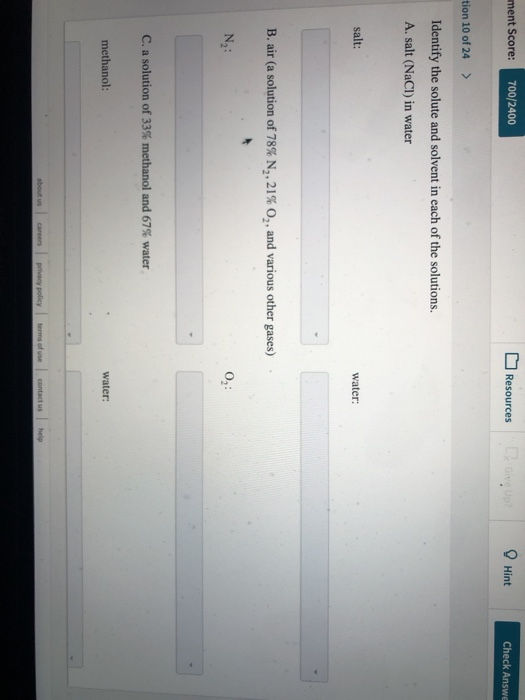700/2400 Up Hint ment Score: Resources Check Answe tion 10 of 24 > Identify the solute and solvent in each of the solutions. A. salt (NaCI) in water salt: water: B. air (a solution of 78% N, , 21% O,, and various other gases) N2: C. a solution of 33% methanol and 67% water methanol: water about us terms of use contact us help careers privacy policy ssignment Score: 700/2400 Resources Hint Check Answer Question 10 of 24 C. a...

• ### 71.1% Resources Give Up? Hint A current of 5.44 A is passed through a Fe(NO3), solution....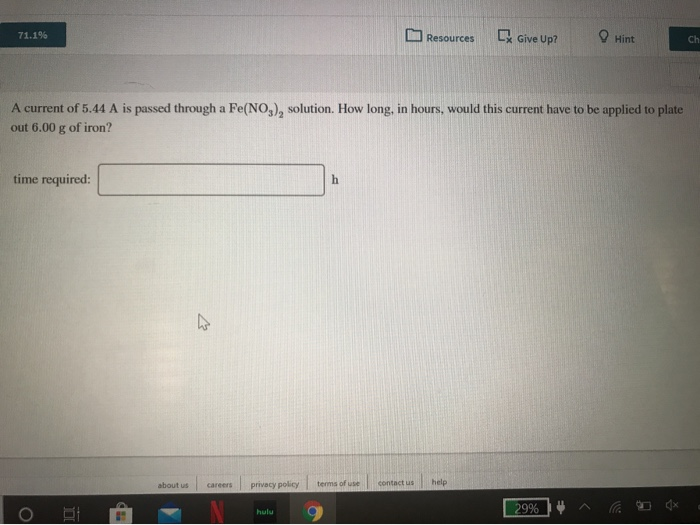71.1% Resources Give Up? Hint A current of 5.44 A is passed through a Fe(NO3), solution. How long, in hours, would this current have to be applied to plate out 6.00 g of iron? time required: about us Careers privacy policy terms of 29% ^ a *

• ### Calculate the molarity of the two solutions. a) 0.650 mol of NaOH in 2.65 L of...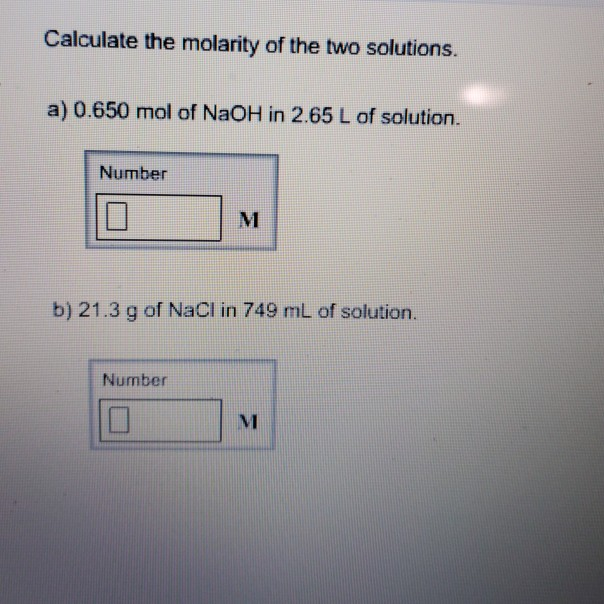Calculate the molarity of the two solutions. a) 0.650 mol of NaOH in 2.65 L of solution. Number b) 21.3 g of NaCI in 749 mL of solution. Number

Free Homework App